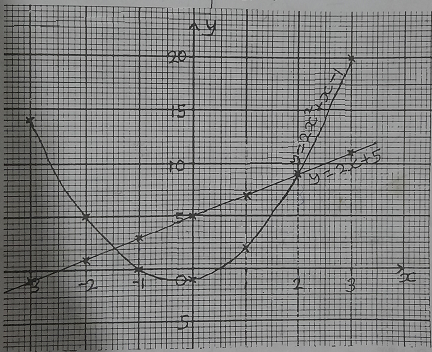mycollegehive
If x = -2.5, what is the value of y on the curve?
waec-2020 ssce-exam-2020 math waec-mathematics-20200The graphs of the equations y = 2x + 5 and y = 2x2 + x - 1 are shown.

if x = -2.5, what is the value of y on the curve?

A. y = 8.0

B. y = 8.5

C. y = 9.0

D. y = 9.5

171 viewsShareFollowSchool not set Nigeria
19 August 2020University of Lagos Nigeria
15 September 20200y = 8.5

Ans BShare### Related Tags

waec-2020

8 followers

273 questionsssce-exam-2020

6 followers

273 questionsmath

9 followers

1018 questions